## Abstract

This paper analyzes Chinese household CO2 emissions in 1994–2012 based on the Logarithmic Mean Divisia Index (LMDI) structure decomposition model, and discusses the relationship between household CO2 emissions and economic growth based on a decoupling indicator. The results show that in 1994–2012, household CO2 emissions grew in general and displayed an accelerated growth trend during the early 21st century. Economic growth leading to an increase in energy consumption is the main driving factor of CO2 emission growth (an increase of 1.078 Gt CO2 ) with cumulative contribution rate of 55.92%, while the decline in energy intensity is the main cause of CO2 emission growth inhibition (0.723 Gt CO2 emission reduction) with cumulative contribution rate of 38.27%. Meanwhile, household CO2 emissions are in a weak state of decoupling in general. The change in CO2 emissions caused by population and economic growth shows a weak decoupling and expansive decoupling state, respectively. The CO2 emission change caused by energy intensity is in a state of strong decoupling, and the change caused by energy consumption structure fluctuates between a weak and a strong decoupling state.

## Keywords

Household energy consumption ; CO2 emissions ; LMDI model ; Decoupling indicator

## 1. Introduction

In 1896, Swedish scientist Svante Arrhenius mentioned that CO2 emissions could lead to global climate warming (Arrhenius, 1896 ). With economic development, industrialization becomes an inevitable route for every country. The series of problems caused by CO2 emissions have become more and more important, and draw a great deal of attention from society. In 2013, the IPCC Fifth Assessment Report (IPCC, 2013 ) pointed out that human activity has been the dominant cause of observed warming since 1950, and the certainty is up to 95%. Meanwhile, in the past 130 years, global temperature has risen by 0.85 °C, and in the future, big cities will be the main source of CO2 emissions, caused by human activities.

China has achieved the goal of rapid economic growth since its Reform and Opening-up. However, as the economy grows, China has surpassed the U.S. to become the biggest carbon emitter in 2013 (Friedlingstein et al., 2014 ). In China, the proportion of energy consumption in the industrial sector has remained at more than 80%, which is the main reason why Chinas energy consumption and CO2 emissions are growing rapidly. Hence, policies and researches on the energy crisis and CO2 emission control are mainly focused on the industrial sector, ignoring the household sector, which is at the lower end of energy consumption. With urbanization, a tremendous change has occurred in the lifestyle of Chinas citizens, and household energy consumption has become an important part of total energy consumption. Chinas household energy consumption has maintained growth for the past 20 years and presents an accelerating growth trend during the 21st century (NBSC, 2013 ).

Linden and Annika (2002) pointed out that in northern Europe, household energy consumption accounts for more than 20% of the total energy consumption. This phenomenon is particularly prominent in Sweden where 38% of the energy consumption comes from housing sector. With urbanization and economic rates continuing to grow rapidly, the quality of life in China will go on improving. Hence, we can see that household energy consumption will account for more of the total energy consumption and become another important factor in energy consumption growth in the future.

The household CO2 emission is closely associated with the household energy consumption from urban and rural residents. In recent years, as household energy consumption and CO2 emission have grown at an increasing rate, more and more scholars began to pay attention to these issues (Wang and Shi, 2009 , Papathanasopoulou, 2010 , Feng et al., 2011a , Feng et al., 2011b , Liu et al., 2011 , Liu and Sweeney, 2012 , Hamamoto, 2013  and Jones and Kammen, 2014 ). Lenzen (1998) applied an input–output model to calculate household greenhouse gas (GHG) emissions in Australian; the results showed that increased household consumption is the main reason for the growth of GHG emissions, and that the amount is much larger than the emission reduction resulting from technological progress and industrial upgrading. Zhao and Li (2011) illustrated the major drivers behind Chinas household energy consumption based on Logarithmic Mean Divisia Index (LMDI) model, and found that the increase in household purchasing power and the shifts to energy-intensive products played the most important roles in household energy consumption.

The decoupling indicator has been widely used to evaluate the relationship between resource consumption and economic growth. OECD (2002) put forward the concept of the decoupling indicator, which measures the decoupling state by the ratio of the environment pressure growth rate to the driving force growth rate. Tapio (2005) and Tapio et al. (2007) defined further details of the decoupling state, and studied the decoupling relationship between CO2 emissions and economic growth in the transport departments of 15 European countries during 1970–2001. The research found that none of these countries' CO2 emissions decreased as expected and these 15 countries are finding it difficult to achieve the goal set in the Kyoto Protocol to reduce CO2 emissions by 8% during 2008–2012. Lu et al. (2011) formulated a pair of new decoupling indicators based on the IGT equation, related to resource use, and the Ie GTX equation, concerned with waste emissions. They analyzed the decoupling state of energy use and SO2 emission in the U.S. and China between 2000 and 2007, and found that both decoupling indicators for energy use and SO2 emission in China were slightly lower than that in the U.S.

It can be concluded that, with economic growing, CO2 emissions caused by household consumption have become more and more important. Identifying the key impacts of household CO2 emission growth and analyzing the decoupling relationship between economic growth and CO2 emissions will play an important role in energy saving and emission reduction. In this paper, we analyze the factors of Chinas household CO2 emissions in 1994–2012 based on the LMDI (Ang and Lee, 1994 , Ang and Zhang, 2000  and Ang, 2005 ) structure decomposition model, and discuss the relationship between household CO2 emissions and economic growth based on the decoupling indicator.

The remainder of this paper is organized as follows: Section 2 presents the LMDI model and the decoupling indicators of Chinas household CO2 emissions. Section 3 discusses the related data sources and process. Section 4 presents the main result. The conclusion and suggestions are summarized in Section 5 .

## 2. Methodology

### 2.1. LMDI model formulation of Chinas household CO2 emissions

According to the emission factor approach given by IPCC (2006) , we chose coal products, oil products, gas, electricity, heat, and other energy types as the household energy sources. Total CO2 emissions from household consumption can be expressed as follows:

 $C={\sum }_{i}C_{i}={\sum }_{i}E_{i}\times c_{i}.$
( 1)

From the existing research (Xu et al., 2006 , Guo, 2010 , Wang, 2012  and Ma et al., 2015 ), we know that direct household CO2 emissions can be affected by economics, energy structure, population, and energy intensity. According to the Kaya identity, we can decompose CO2 emissions into four effects as follows: population effect (${\textstyle C_{\mbox{pop}}}$ ), economic activity effect (${\textstyle C_{\mbox{act}}}$ ), energy intensity effect (${\textstyle C_{\mbox{int}}}$ ), and energy structure effect (${\textstyle C_{\mbox{str}}}$ ) as follows:

 $C={\sum }_{i}C_{i}={\sum }_{i}P{\frac {Y}{P}}{\frac {E}{Y}}{\frac {E_{i}}{E}}{\frac {C_{i}}{E_{i}}}=$${\sum }_{i}PyIe_{i}c_{i}.$
( 2)

The variables are used is defined: ${\textstyle i}$ represents energy type; ${\textstyle E}$ represents household energy consumption and ${\textstyle E_{i}}$ represents household energy consumption for energy type ${\textstyle i}$ ; ${\textstyle P}$ and ${\textstyle Y}$ stand for population and GDP respectively; ${\textstyle y}$ is defined as GDP per capita, y  = Y /P   ; ${\textstyle I}$ is defined as energy intensity, I  = E /Y   ; ${\textstyle e_{i}}$ represents proportion of energy consumption for energy type ${\textstyle i}$ in household energy consumption; ${\textstyle C_{i}}$ represents CO2 emissions for energy type ${\textstyle i}$ , and ${\textstyle c_{i}}$ represents CO2 emission factor for energy type ${\textstyle i}$ , ci  = Ci /Ei . Each effect can be calculated as follows:

 $\Delta C_{\mbox{tot}}=C^{t}-C^{0}=\Delta C_{\mbox{pop}}+$$\Delta C_{\mbox{act}}+\Delta C_{\mbox{int}}+\Delta C_{\mbox{str}}{\mbox{;}}$
( 3)

 $\Delta C_{\mbox{pop}}=\sum _{i}{\frac {C_{i}^{t}-C_{i}^{0}}{ln\quad C_{i}^{t}-ln\quad C_{i}^{0}}}ln\left({\frac {P^{t}}{P^{0}}}\right){\mbox{;}}$
( 4)

 $\Delta C_{\mbox{act}}=\sum _{i}{\frac {C_{i}^{t}-C_{i}^{0}}{ln\quad C_{i}^{t}-ln\quad C_{i}^{0}}}ln\left({\frac {y^{t}}{y^{0}}}\right){\mbox{;}}$
( 5)

 $\Delta C_{\mbox{int}}=\sum _{i}{\frac {C_{i}^{t}-C_{i}^{0}}{ln\quad C_{i}^{t}-ln\quad C_{i}^{0}}}ln\left({\frac {I^{t}}{I^{0}}}\right){\mbox{;}}$
( 6)

 $\Delta C_{\mbox{str}}=\sum _{i}{\frac {C_{i}^{t}-C_{i}^{0}}{ln\quad C_{i}^{t}-ln\quad C_{i}^{0}}}ln\left({\frac {e^{t}c^{t}}{e^{0}c^{0}}}\right){\mbox{.}}$
( 7)

### 2.2. Decoupling indicator of household CO2 emissions and economic growth

The decoupling indicator is used to measure the correlation between indicators. A decoupling state means that the growth rate of CO2 emissions is less than that of the GDP. In this paper, we analyze the decoupling state effects of population, economic activity, energy intensity, and energy structure, derived from the LMDI model, as follows:

 $D_{r}={\frac {\%\Delta C_{\mbox{tot}}}{\%\Delta {\mbox{GDP}}}}$ $={\frac {\%\Delta C_{pop}+\%\Delta C_{act}+\%\Delta C_{int}+\%\Delta C_{str}}{\%\Delta {\mbox{GDP}}}}$ $=D_{r}^{\mbox{pop}}+D_{r}^{\mbox{act}}+D_{r}^{int}+D_{r}^{\mbox{str}}{\mbox{,}}$
( 8)

In equation (8), ${\textstyle D_{r}}$ is the total decoupling indicator, ${\textstyle D_{r}^{\mbox{pop}}}$ , ${\textstyle D_{r}^{\mbox{act}}}$ , ${\textstyle D_{r}^{int}}$ , ${\textstyle D_{r}^{\mbox{str}}}$ represent the decoupling indicators of the four effects, ${\textstyle \%\Delta {\mbox{GDP}}}$ is the GDP growth rate, and ${\textstyle \%\Delta C}$ is the reduction rate of CO2 emissions.

According to economics, CO2 emissions, and ${\textstyle D_{r}}$ , Tapio (2005) defined eight decoupling states, as shown in Fig. 1 .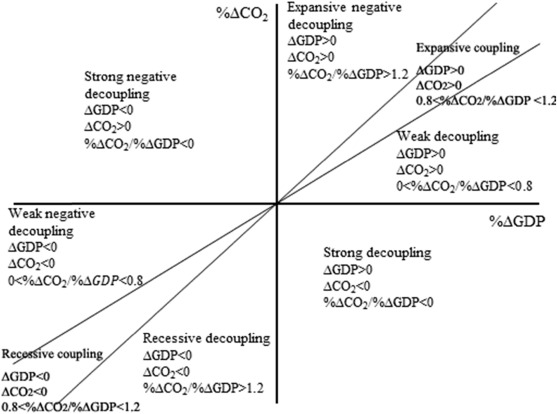Fig. 1. The degree of coupling and decoupling of CO2 emission reduction from economic growth. The two lines represent that Dr  = 0.8 or 1.2 and the states are both coupling (modified from Tapio, 2005 ).

Over the past two decades, Chinas GDP has kept growing rapidly. Therefore, we discuss the situation where ${\textstyle \Delta {\mbox{GDP}}>0}$ . The GDP growth rate and CO2 emissions can be coupled, strong decoupled, weak decoupled or negatively decoupled. Strong decoupling occurs when ${\textstyle D_{\mbox{r}}<0}$ , and CO2 emissions decrease with economic growth. Weak decoupling occurs when ${\textstyle 0 , meaning that CO2 emissions increase with economic growth, but the growth rate is less than that of the economy. Negative decoupling occurs when ${\textstyle D_{\mbox{r}}>1.2}$ , meaning that CO2 emissions increase with economic growth, and that the growth rate is higher than that of the economy. Coupling occurs when ${\textstyle 0.8 , meaning that the growth rate of CO2 emissions is equal to the growth rate of the economy.

## 3. Data description

The research period in this paper is 1994–2012. The household CO2 emissions considered include those caused by direct household energy consumption in terms of lighting, cooking, heating, household appliances, and private transportation. Electricity consumption belongs to household direct energy consumption, so the CO2 emitted by electricity consumption belongs to household direct CO2 emissions. Data on all kinds of fuel consumption, GDP, and population were collected from the China Statistical Yearbook (NBSC, 2014 ) and the China Energy Statistical Yearbook (NBSC, 2013 ). Conversion factors from physical units to coal equivalent were obtained from the China Energy Statistical Yearbook 2013 Appendix IV. Emission factors (except electricity) were collected from Jin and Wu (2013) for reference. The emission factor for electricity (EEF) is calculated as follows:

 ${\mbox{EEF}}=\sum {\mbox{EGF}}\times {\mbox{NCR}}\times {\mbox{SSG}}\times {\mbox{FCEF}},$
( 9)

In equation (9), EGF is the percentage of electricity generated from fossil-fuels, NCR is the net coal consumption rate for fossil-fuel power plants, SSG is the source of fossil-fuel power generation, and FCEF is fossil-fuel CO2 emission factors. If there is 1 kW h of electricity produced, the CO2 emission is EEF. ${\textstyle {\mbox{EGF}}\times {\mbox{NCR}}}$ is the standard coal consumption of 1 kW h electricity. The standard coal consumption of 1 kW h electricity times the proportion of each energy consumption rate and its emission factor is EEF (Table 1) .

Table 1. Data for calculating CO2 emission factor of electricity.
EGF (%) NCR (kg ce (kW h)−1 ) SSG (%) EEF (kg CO2 (kW h)−1 )
Coal Oil Gas Others
79.00 0.305 94.3 0.5 2.3 2.9 0.6406

Electricity and heat are the secondary energy. The composition of electricity mainly includes thermal power, hydropower, nuclear power and wind power. Little carbon is emitted in hydropower, nuclear power and wind power, so they are not be considered. However, CO2 is emitted in thermal power in the electricity production which directly consumes coal, oil, gas and other energy. Thus, the CO2 emissions of electricity are calculated by extracting and decomposing all kinds of fossil energy consumptions. Similarly, the CO2 emissions of heat are calculated by decomposing all kinds of fossil energy consumptions (Wang, 2012 ). Because various fossil energy consumption data directly used in heating department are not available and the total heat consumption of residents is small, the CO2 emissions of heat are not considered. A summary of various types of energy CO2 emission factors and integrated units is shown in Table 2 .

Table 2. Emission factors for every energy type (kg CO2 (kg ce)−1 ).
Energy type Coal Coke Crude oil Gasoline Kerosene Diesel oil Burner oil Gas Electricity Other energy
Emission factor 2.7687 3.1339 2.0929 2.0284 2.0929 2.1681 2.1363 1.6423 5.2127 1.6267

## 4. Results and discussion

### 4.1. Direct household CO2 emissions decomposition analysis based on LMDI model

During 1994–2012, Chinas household CO2 emissions decreased slightly at first, then grew rapidly. During this period, CO2 emissions increased by 426 Mt. A U-shape curve is presented in Fig. 2 .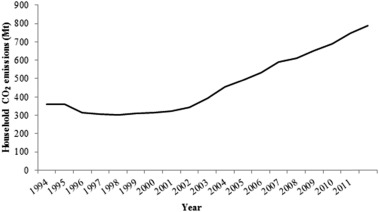Fig. 2. Direct household CO2 emissions during 1994–2012 in China.

According to Eqs. (1) , (2) , (3) , (4) , (5) , (6)  and (7) in Section 2 , the components of the decomposition analysis can be shown in Fig. 3 . The economic activity effect is the main driving factor for the household CO2 emission increasing, while the decline in energy intensity is the main inhibition of the increasing. The energy structure effect is responsible for decreased CO2 emissions and the population effect is responsible for increased emissions. The effects of energy structure and population both are weak.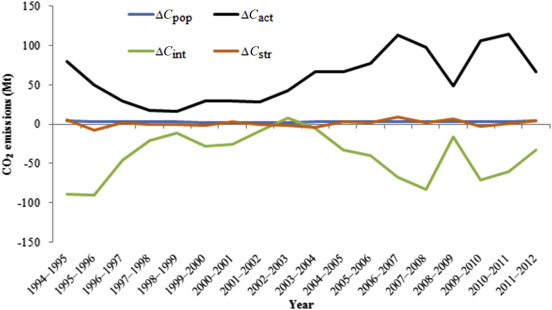Fig. 3. LMDI decomposition results during 1994–2012. ${\textstyle \Delta C_{\mbox{pop}}}$ , ${\textstyle \Delta C_{\mbox{act}}}$ , ${\textstyle \Delta C_{int}}$ , ${\textstyle \Delta C_{\mbox{str}}}$ present population effect, economic activity effect, energy intensity effect and energy structure effect, respectively.

We can see from Fig. 4 that economic activity effect plays a dominant role, accounting for a 55.92% change in CO2 emissions in absolute values. In 2003–2004, the maximum value of the contribution rate of economic activity effect was 83.73%; the minimum value of 32.79% was in 1995–1996. The energy intensity effect had a total contribution rate of 38.27%, which is the second largest total contribution rate during the research period; it shows a large fluctuation, and the contribution rate is positive except for 2002–2003. In particular, the contribution rate of the energy intensity effect (49.94%, 59.91%, 56.87%, 48.72%) is even higher than the economic activity effect (45.11%, 32.79%, 36.44%, 41.91%) in 1994–1998. Contribution rates of the energy structure and population structure effects are much lower at 3.11% and 2.70%, respectively.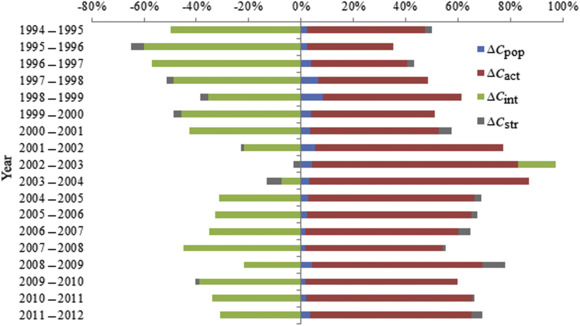Fig. 4. Contribution rates for population effect, economic activity effect, energy intensity effect and energy structure effect rate during 1994–2012.

Economic growth is the main reason for the increase in CO2 emissions. Chinas economic level has increased rapidly since Reform and Opening-up; the per capita GDP increased from CN¥ 4021.5 in 1994 to CN¥ 38,325.5 in 2012, and the average annual growth rate reached 12.58%. With the increase in income, demands for the comforts in life and traffic convenience become higher and higher, leading directly to increases in energy consumption and CO2 emissions.

The increase in CO2 emission caused by economic growth is found to reach 1.078 Gt during 1994–2012, 2.53 times the actual CO2 emission increase. The household CO2 emission increase caused by economic growth shows a similar changing trend to the GDP growth rate (Fig. 5 ).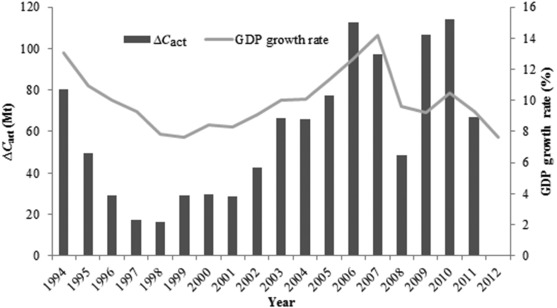Fig. 5. The variation of ${\textstyle \Delta C_{\mbox{act}}}$ and GDP growth rate during 1994–2012.

CO2 emissions from the economic activity effect are directly linked to the GDP growth rate. Currently China is in the process of industrialization and urbanization, and economic development is still the primary factor driving these developments. In the future, the economic activity effect will still be the main driving factor for CO2 emission increase.

Energy intensity is a measure of the energy efficiency of a nations economy. It is calculated as units of energy consumed per unit of GDP. Energy intensity effect is the key factor that delays CO2 emission growth. With improvements in technology, energy efficiency is increasing, so household energy intensity is declining. During 1994–2012, household energy intensity decreased from 27.1 tce to 5.4 tce per million CN¥. The growth rates of energy intensity were negative except for 2002 (Fig. 6 ). The decrease in CO2 emission caused by the energy intensity decrease reached 0.723 Gt. So, developing energy-saving and emission-reduction technologies, and improving household energy efficiency will be the most effective ways to control household CO2 emissions.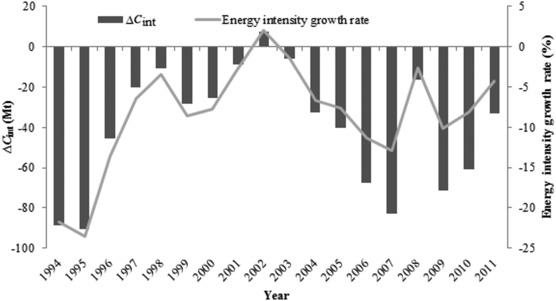Fig. 6. The variation of ${\textstyle \Delta C_{int}}$ and energy intensity growth rate during 1994–2012.

Energy structure effect is another important factor for CO2 emissions. Fig. 7 displays the proportion of five main types of energy consumption. Coal is the major energy source in China. The proportion of coal in total energy consumption keeps decreasing but still remains at more than 70%, and coal is still the most important energy source for household consumption.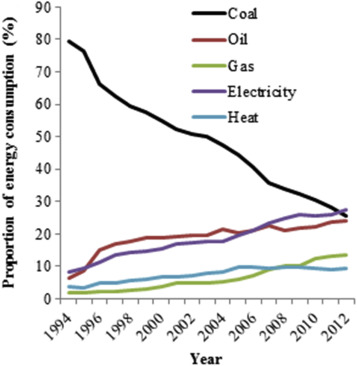Fig. 7. Energy consumption structure during 1994–2012 in China.

A change in coal consumption is the main factor leading to CO2 emission reduction from the energy structure effect. ${\textstyle \Delta C_{{\mbox{str}}1}}$ was negative during the research period, contributing 0.229 Gt household CO2 emission reduction (Fig. 8 ). Meanwhile, household energy demand for oil, gas, and electricity is growing rapidly. Oil and gas contribute 0.087 Gt to CO2 emission increase, and electricity contributes 0.166 Gt. The proportion of electricity generation from coal in China (79%) (NBSC, 2013 ) is much higher than the world average (41.3%) in 2012, which is the main factor leading to Chinas high CO2 emissions per unit of electricity.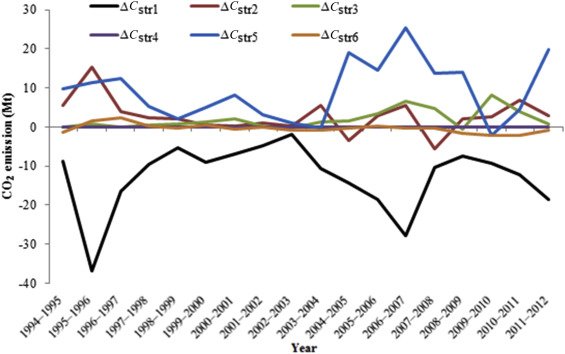Fig. 8. Energy structure effect for each energy type during 1994–2012 (${\textstyle \Delta C_{{\mbox{str}}1}}$ , ${\textstyle \Delta C_{{\mbox{str}}2}}$ , ${\textstyle \Delta C_{{\mbox{str}}3}}$ , ${\textstyle \Delta C_{{\mbox{str}}4}}$ , ${\textstyle \Delta C_{{\mbox{str}}5}}$ , ${\textstyle \Delta C_{{\mbox{str}}6}}$ present coal, oil, gas, electricity, heat, and other energy, respectively).

It can be concluded that there is a huge potential for CO2 emission reduction in the energy structure. Adjusting the energy structure, using solar energy, wind energy, and other clean energy to replace traditional energy, adjusting the structure of Chinas electricity generation, and reducing the number of small thermal power station will be important for limiting CO2 emissions.

Chinas population increased from 1.1985 billion to 1.354 billion during 1994–2012, with the average growth rate of 0.64%. We can see from the decomposition result that population has a positive effect, but the effect is weak and gentle.

### 4.2. Decoupling economic growth from direct household CO2 emissions

Economic growth is the main driving factor for the growth in CO2 emissions based on the LMDI model. So what is the decoupling relationship between economic growth and CO2 emissions? We can get the result according to Tapios decoupling indicator model (Fig. 9 ).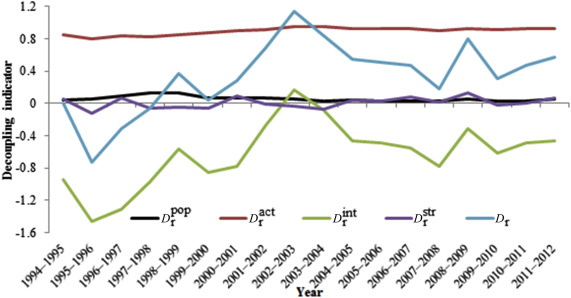Fig. 9. Decoupling indicator for household CO2 emissions during 1994–2012. ${\textstyle D_{r}^{\mbox{pop}}}$ , ${\textstyle D_{r}^{\mbox{act}}}$ , ${\textstyle D_{r}^{int}}$ , ${\textstyle D_{r}^{\mbox{str}}}$ , ${\textstyle D_{r}}$ present decoupling indicator for population effect, economic activity effect, energy intensity effect energy structure effect and total effect, respectively.

During the research period, the decoupling indicator was 0–0.8 for most years. Household CO2 emissions present weak decoupling with economic growth, which means that CO2 emission increase with economic growth, but the growth rate is lower than the economic growth rate. During 1995–1998, the decoupling indicator was negative, and CO2 emissions were in a state of strong decoupling. This means that the inhibition effect of CO2 emissions played a key role during this period and that the CO2 emissions declined, while the economy grew. However, during 2002–2004 and 2008–2009, the decoupling indicator was between 0.8 and 1.2, and it presented a state of coupling for CO2 emissions and economic growth, which were basically synchronized.

The decoupling indicator for the population effect ${\textstyle D_{r}^{\mbox{pop}}}$ was 0–0.8 for the whole research period, this means that the CO2 emission growth rate caused by the population effect was lower than the economic growth rate. Its average contribution rate was 3.48%. The value of ${\textstyle D_{r}^{\mbox{str}}}$ fluctuates around 0 and the decoupling state changes between strong and weak. The average contribution rate of the decoupling indicator for the energy structure effect is 3.32%. The decoupling indicator for the energy intensity effect is negative for most years. This means that the decline in energy intensity promoted the decoupling of CO2 emissions and economic growth. The average contribution rate is 34.66%. The value of ${\textstyle D_{r}^{\mbox{act}}}$ maintained at ${\textstyle >0.8}$ and is in a state of coupling. The average contribution rate is 56.95%.

The decoupling indicator of each energy type for household CO2 emissions is calculated. The results are shown in Fig. 10 . We can see from the result that the decoupling indicators for coal and electricity are key factors in the energy structure effect. The decline in CO2 emissions, caused by a decrease in coal consumption, is higher than the CO2 emission growth caused by electricity consumption increase, for the years when it is in a state of strong decoupling. From this we can see that CO2 emission increase from electricity is an important factor for the inhibition of CO2 emissions overall, and that economic growth enters a state of strong decoupling.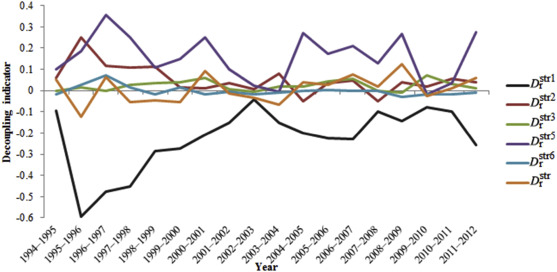Fig. 10. Decoupling indicator for each energy type during 1994–2012 (${\textstyle D_{\mbox{r}}^{{\mbox{str}}1}}$ , ${\textstyle D_{\mbox{r}}^{{\mbox{str}}2}}$ , ${\textstyle D_{\mbox{r}}^{{\mbox{str}}3}}$ , ${\textstyle D_{\mbox{r}}^{{\mbox{str}}5}}$ , ${\textstyle D_{\mbox{r}}^{{\mbox{str}}6}}$ present decoupling indicator for coal, oil, gas, electricity, and other energy type respectively. ${\textstyle D_{\mbox{r}}^{{\mbox{str}}4}}$ which presents decoupling indicator for heat is too small, so data are not displayed).

During the research period, the decoupling of the direct household CO2 emissions and economic growth exhibited the following four states, showing the change process: strong decoupling‒weak decoupling‒coupling‒weak decoupling. Combined with Fig. 9 , it is found that the fluctuations in the economic, population, and energy structure effects are very gentle, but that the energy intensity effect has strong volatility. This resulted in the changing trend of the total decoupling indicator and the decoupling indicator of the energy intensity effect is basically synchronized. Therefore, we can deduce that Chinas direct household CO2 emissions may remain in a state of weak decoupling for a few years. Improving energy-saving technologies would be the most efficient way to promote CO2 emissions and economic growth change decoupling from weak to strong.

## 5. Conclusions

In this paper, the factors in Chinas household direct CO2 emissions based on a LMDI model are analyzed, and the decoupling state of CO2 emissions based on Tapios decoupling indicator model are discussed. The main conclusions drawn from this study may be summarized as follows:

• During 1994–2012, Chinas household CO2 emissions decreased slightly at first, and then grew rapidly. And the household CO2 emissions increased by 426 Mt in 2012 compared to that in 1994.
• The economic activity effect is the key factor for CO2 emissions with cumulative contribution rate of 55.92%. CO2 emission caused by economic activity is direct related to GDP growth rate.
• The energy intensity effect is the main factor for restraining CO2 emission growth, and the CO2 emission decrease is relatively synchronized to the energy intensity growth rate. During 1994–2012, household energy intensity decreased from 27.1 tce to 5.4 tce per million CN¥. The decrease in CO2 emission caused by the energy intensity decrease reached 0.723 Gt.
• There has been a big structural change in household energy sources, where the change in coal consumption is the main reason for the decline in CO2 emissions (reduction of 0.229 Gt). The change in electricity consumption has had the opposite effect (an increase of 0.166 Gt).
• The population effect leads to CO2 emissions increasing, but the effect is weak (lower than 8.5%).
• During the research period, the household CO2 emissions were generally in a weak state of decoupling, but showed a strong decoupling during 1995–1998, and coupling for 2002–2004 and 2008–2009.
• Decoupling indicators for the economic activity and population effects are positive, and are in the state of coupling and weak decoupling for two effects. The decoupling indicator for the energy intensity effect is negative and in a state of strong decoupling. The value of the decoupling indicator for the energy structure effect fluctuates around 0. That is to say, energy structure effect changes between the states of strong decoupling and weak decoupling.
• There are four states for the decoupling of CO2 emissions, which show the change process as follows: strong decoupling‒weak decoupling‒coupling‒weak decoupling. The changing trend of the total decoupling indicator and the energy intensity effect decoupling indicator are basically synchronized.

To control direct household CO2 emissions with economic growth and to promote the decoupling effect, following policy recommendations are put forward based on the main conclusions:

• China is in the process of industrialization and urbanization, and the GDP growth rate will maintain its high level in the future. Demands for good housing, personal vehicles, and household appliances are at a peak. Meanwhile, direct household CO2 emissions will remain in a weak decoupling state, and CO2 emissions will still increase with economic growth. Therefore, it is important to promote proper consumption patterns and concepts, and to aspire an energy-saving and emission-reducing lifestyle.
• Decreasing energy intensity is the main driving factor to reduce CO2 emission growth, and technological improvement is the key to decreasing energy intensity. Therefore, development of low-carbon and highly energy-efficiency technologies will be the most effective way to promote a change in household CO2 emissions from a weak to a strong decoupling state.
• Coal still makes up the largest proportion of household energy consumption. Optimizing the energy structure by promoting natural gas and other clean energies will contribute to the upgrading of Chinas household energy consumption structure. Meanwhile, with the consumption of electricity continuing to increase CO2 emission problems, caused by Chinas electricity generation structure of primarily thermal power, are becoming increasingly prominent. Therefore, adjusting Chinas electricity generation structure with increasing proportions of gas, hydro, and nuclear power will help control direct household CO2 emissions.

## Acknowledgements

This work was supported by the National Natural Science Foundation of China (NSFC) under Grant No. 71573015 , 71303019 , 71173206 , and 71521002 .

### Document informationPublished on 15/05/17
Submitted on 15/05/17

Licence: Other

### Document Score0

Views 27
Recommendations 0